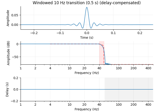# mne.time_frequency.morlet#

mne.time_frequency.morlet(sfreq, freqs, n_cycles=7.0, sigma=None, zero_mean=False)[source]#

Compute Morlet wavelets for the given frequency range.

Parameters:
sfreq`float`

The sampling Frequency.

freqs`array`

Frequency range of interest (1 x Frequencies).

n_cycles`float` | `array` of `float`, default 7.0

Number of cycles. Fixed number or one per frequency.

sigma`float`, default `None`

It controls the width of the wavelet ie its temporal resolution. If sigma is None the temporal resolution is adapted with the frequency like for all wavelet transform. The higher the frequency the shorter is the wavelet. If sigma is fixed the temporal resolution is fixed like for the short time Fourier transform and the number of oscillations increases with the frequency.

zero_meanbool, default `False`

Make sure the wavelet has a mean of zero.

Returns:
Ws

The wavelets time series.

## Examples using `mne.time_frequency.morlet`#Background information on filtering

Background information on filtering